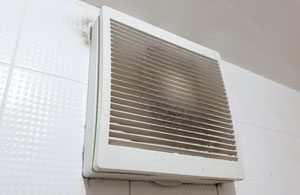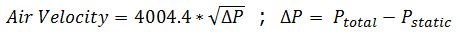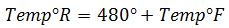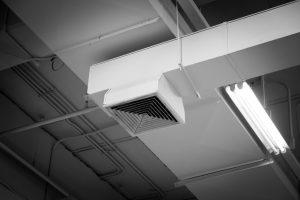# Velocity Measurement and Environmental EffectsDifferential pressure measurement can be beneficial in a wide variety of building automation system (BAS) applications.

One of these applications involves determining if a filter in your system needs to be cleaned or replaced. Filter health can be established by monitoring the pressure on the primary and secondary sides of a filter. A clean filter will typically have a baseline differential pressure generated as air flows through it. As the filter becomes dirty, this differential pressure increases.

When using a mechanical flow sensor, such as a pitot tube, differential pressure can also be used to determine flow velocity and volumetric flow.

Pitot tubes are used to measure static pressure and total pressure.  By subtracting the static pressure of a system from the total pressure, a differential pressure is generated. The standard equation for calculating velocity in feet per minute (FPM) is as follows:The units of pressure for this example are in inches of water column. The equation above is based on standard temperature and density. The 4004.4 constant is derived from standard conditions set at 29.92 in Hg atmospheric pressure and absolute standard temperature 530°R. Absolute Rankine temperature is derived as follows:In this example, the standard temperature used for our 4004.4 constant in Fahrenheit is 70°F.

What does all of this mean? The environment in which flow measurements are monitored will rarely be taken under standard conditions. In order to ensure accurate velocity measurements, corrections must be applied to the standard equation.

Our Pitot Tube, Series 160, includes a simple equation in its instruction manual, which is similar to the standard equation above, but takes air density into account in pounds per cubic foot (PCF).  This simple equation is as follows:Now let’s bring it all together into one example:

Temp = 65°F
Barometric Pressure = 30.29 inHg
∆P = 0.5″ w.c.

First, we will calculate the velocity without correcting for air density.Next, we’ll solve for the same differential pressure, but corrected for air density.These yield a difference of 24.84 ft/min. If we consider volumetric flow rate in a 3 foot diameter duct, this would cause a difference of 175.58 CFM. This may be extremely important depending on what the volumetric flow is being used for, such as reporting emissions information.In conclusion, differential pressure can be useful in determining an accurate air velocity. By following our equation, we’re able to adjust for environmental conditions. This is especially important in applications where a high accuracy is necessary.